Tire Model in Driving Simulator

The primary forces during lateral maneuvering, acceleration, and braking are generated by tires as a function of the driver input. The linear analysis of a tire model commonly considers constant tire side force coefficients at small force output range. The linear tire model doesn't consider longitudinal tire forces due to the complex interactions between lateral and longitudinal tire forces. Thus, linear tire model is suitable for analyzing a stable vehicle behavior under the assumption of small steering and acceleration. Most of AHS (Automated Highway System) utilize the linear tire model for its simplicity. In our driving simulator, it is very important to describe the exact behavior of a vehicle in any driving scenario including inclement driving conditions which may require severe steering, braking, acceleration, and other driving related operations. Therefore, in order to simulate the complete vehicle operational range, it is important to properly model tire forces containing the interactions of longitudinal and lateral forces from small levels through saturation. The tire model used in the driving simulator is based on a paper from U.S Department of Transportation . The paper presents complete computations of the tire forces by using the parameters available from various tire test results including comprehensive Calspan model and data. The tire model developed in this paper provides a useful force producing element for a full driver/vehicle model as in our driving simulator. In the following sections, physical and analytical tire model is presented with basic tire variables followed by the identified model equations and resulting plots. Especially, the tire force plots provide not only the validation of this tire model, but also insights into tire effects on vehicle response and stability.

Slip Angle and Longitudinal Slip

The longitudinal and lateral forces generated by a tire are a function of the slip angle and longitudinal slip of the tire relative to the road.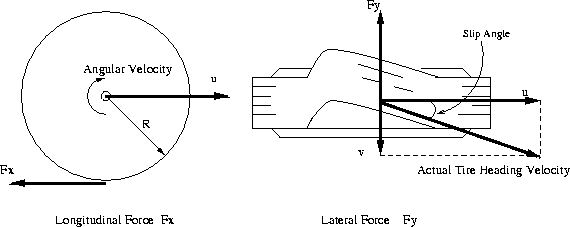Figure 1: Basic Variables of Tire

The longitudinal slip of the tire is defined as a difference between the tire tangential speed and the speed of the axle relative to the road, which is represented by the following equation.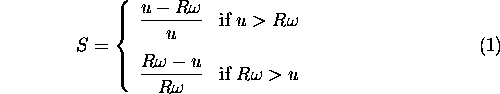where S is the longitudinal slip, R is the radius of the wheel,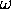is the angular velocity, and u is the speed of the axle illustrated in Figure 1. The value of the longitudinal slip is limited such that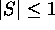. For braking, axle speed is used in the denominator so that longitudinal slip is 1 whenis zero. Slip has the opposite sign when tracking force is generated.
When the tire develops a sideslip velocity denoted by v in Figure 1, a lateral force will develop opposing the sideslip velocity. This lateral force is a function of slip angle, where slip angle is defined as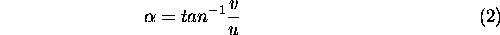where v is the sideslip velocity, and u is the speed of the axle. The value of the slip angle is limited such that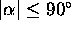.

Simplified Tire Model Equations

The previous theoretical developments lead to a complex, highly non-linear composite force as a function of composite slip. It is convenient to define a saturation function,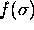, to obtain a composite force with any normal load and coefficient of friction values such thatIn order to derive a simplified computational formulas for our driving simulator, the complex polynomial expression can replaced the complex saturation function with all the considerations in previous section within the agreeable error ranges. The polynomial expression of the saturation function is presented by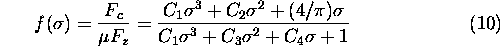where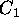,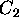,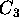, and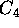are parameters fixed to the specific tires. Table 1 summarizes the tire parameters of 3 different type of tires. RWD bias ply tire model is used for all the driving simulation presented in this paper. The calculation of the composite slip shown in Equation 7 should be modified because the tire contact patch length varies depending on the normal load. The tire contact patch length are calculated using following two equations.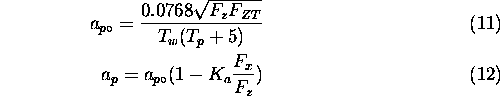whereis the tire contact patch,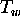is a tread width , and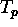is a tire pressure. The values of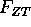andare also shown in Table 1. The lateral and longitudinal stiffness coefficients are a function of tire contact patch length and normal load of the tire as expressed as follows.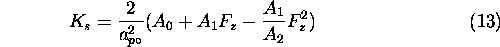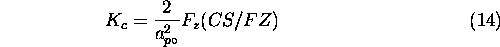where the values of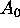,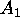,, and CS/FZ are given in Table 1. Then the composite slip calculation becomes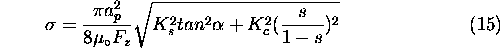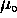is a nominal coefficient of friction and has a value of 0.85 for normal road conditions, 0.3 for wet road conditions, and 0.1 for icy road conditions.Table 1: Parameters for Tire Model Equations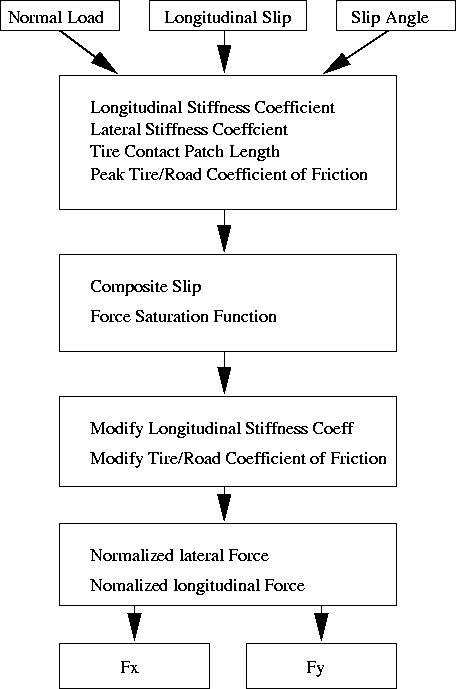Figure 4: Procedures of Tire Force Calculations

Given the polynomial saturation function in Equation 10 and lateral and longitudinal stiffness, the normalized lateral and longitudinal forces are derived by resolving the composite force into the side slip angle and longitudinal slip ratio components.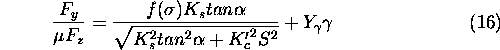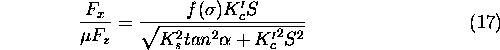lateral force has an additional components due to the tire camber angle,, which is modeled as a linear effect. Under significant maneuvering conditions with large lateral and longitudinal slip, the force converges to a common sliding friction value. In order to meet this criteria, the longitudinal stiffness coefficient is modified at high slips to transition to lateral stiffness coefficient as well as the coefficient of friction defined by the parameter.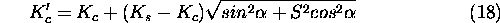The constant value of 0.124 was used forin the tire model. The summarized procedures to calculate the longitudinal and lateral forces are shown in Figure 4. As a result in this section, the longitudinal and lateral tire force plots are included in Figure 5 through Figure 8 for normal driving conditions of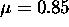.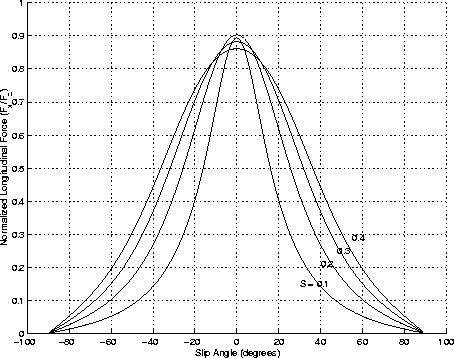Figure 5: Normalized Longitudinal Force vs. Slip Angle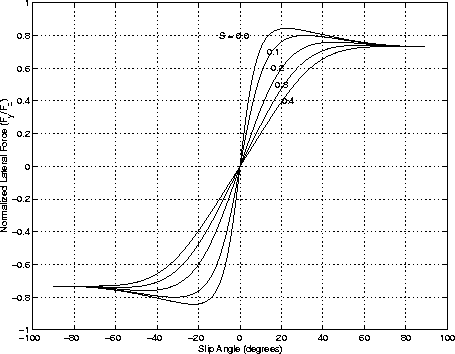Figure 6: Normalized Lateral Force vs. Slip Angle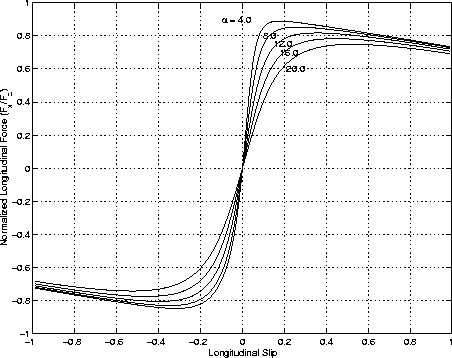Figure 7: Normalized Longitudinal Force vs. Longitudinal Slip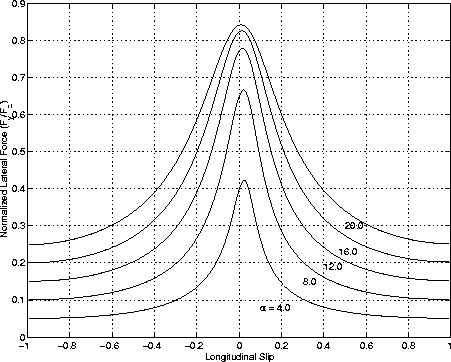Figure 8: Normalized Lateral Force vs. longitudinal Slip

References

1
Szostak, H.T., Allen, W.R., and Rosenthal, T.J., Analytical Modeling of Driver Response in Crash Avoidance Maneuvering Volume II: An Interactive Model for Driver/Vehicle Simulation, U.S Department of Transportation Report NHTSA DOT HS-807-271, April 1988.